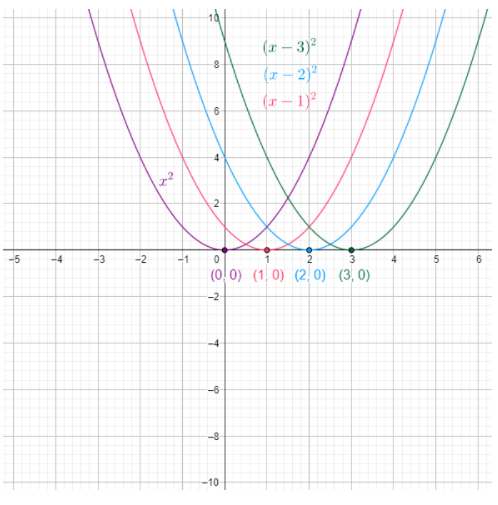# To graph: For the given member of family in viewing rectangle indicated.### Precalculus: Mathematics for Calcu...

6th Edition
Stewart + 5 others
Publisher: Cengage Learning
ISBN: 9780840068071### Precalculus: Mathematics for Calcu...

6th Edition
Stewart + 5 others
Publisher: Cengage Learning
ISBN: 9780840068071

#### Solutions

Chapter 2.2, Problem 70E

(a)

To determine

## To graph: For the given member of family in viewing rectangle indicated.

Expert Solution

### Explanation of Solution

Given information:

The given function is f(x)=(xc)2 .

The value of c is c=0,1,2,3;[5,5]by[10,10] .

Graph:

The graph for the given family of equations is shown in figure (1).Figure (1)

Interpretation: Graph for the family of equations of the function f(x)=(xc)2 is shown in figure (1).

(b)

To determine

Expert Solution

### Explanation of Solution

Given information:

The given function is f(x)=(xc)2 .

The value of c is c=0,1,2,3;[5,5]by[10,10] .

Graph:

The graph for the given family of equations is shown in figure (2).Figure (2)

Interpretation: Graph for the family of equations of the function f(x)=(xc)2 is shown in figure (2).

(c)

To determine

### To find: The conclusions from the graphs and effects on the value of c .

Expert Solution

All the graphs are the same graph but with same y intercepts and different intercepts on x axis.

### Explanation of Solution

Given information:

The given function is f(x)=(xc)2 .

Calculation:

From the graphs in part (a) and (b), we can observed that all the graphs are the same graph with same y intercepts and different intercepts on x axis.

In case when c is less than 0 , then the graph of y=(xc)2 is the same as the graph of y=x2 shifted c units to the left.

In case when c is greater than 0 , then the graph of y=(xc)2 is the same as the graph of y=x2 shifted c units to the right.

Therefore, all the graphs are the same graph but with same y intercepts and different intercepts on x axis.

### Have a homework question?

Subscribe to bartleby learn! Ask subject matter experts 30 homework questions each month. Plus, you’ll have access to millions of step-by-step textbook answers!